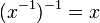# Every group is naturally isomorphic to its opposite group via the inverse map

## Statement

Let$G$ be a group. Then, consider the opposite group of$G$, which is a group with the same underlying set, and such that the binary operation is defined by:$x * y := yx$

In other words, products are taken with order reversed. Then,$G$ is isomorphic to the opposite group via the map$g \mapsto g^{-1}$.

This isomorphism is natural in the sense that it gives a natural isomorphism between the identity functor and the functor sending each group to its opposite group.

## Facts used

1. Inverse map is involutive: This states that$(xy)^{-1} = y^{-1}x^{-1}$ for all$x,y$ in a group, and$(x^{-1})^{-1} = x$ for all$x$ in a group.